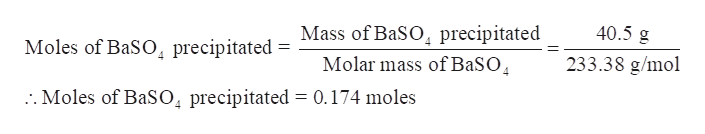# Excess Na2SO4(aq) is added to a 4.97×102 mL sample of industrial waste containing Ba2+ ions. If 40.5 g of BaSO4(s) are precipitated from the reaction, what was the molar concentration of Ba2+ in the original sample?Ba2+(aq)+ Na2SO4(aq) --> BaSO4(s) + 2Na+(aq)

Question
87 views

Excess Na2SO4(aq) is added to a 4.97×102 mL sample of industrial waste containing Ba2+ ions. If 40.5 g of BaSO4(s) are precipitated from the reaction, what was the molar concentration of Ba2+ in the original sample?

Ba2+(aq)+ Na2SO4(aq) --> BaSO4(s) + 2Na+(aq)

check_circle

Step 1

Excess Na2SO4(aq) is added to a 4.97×102 mL sample of industrial waste containing Ba2+ ions. If 40.5 g of BaSO4(s) are precipitated from the reaction, the molar concentration of Ba2+ in the original sample is to be calculated.

Step 2

Write a chemical equation showing reaction between Na2SO4 and Ba2+ ions in a solution.

Step 3

Calculate the moles of BaSO4 precipitated -

Mass of BaSO4 precipitated = 40.5 ...help_outlineImage TranscriptioncloseMass of BaSO precipitated Molar mass of BaSO 40.5 g Moles of BaSO precipitated 233.38 g/mol :. Moles of BaSO, precipitated = 0.174 moles fullscreen

### Want to see the full answer?

See Solution

#### Want to see this answer and more?

Solutions are written by subject experts who are available 24/7. Questions are typically answered within 1 hour.*

See Solution
*Response times may vary by subject and question.
Tagged in

### General Chemistry